# More about Classes Andy Wang Object Oriented Programming

• Slides: 61More about Classes Andy Wang Object Oriented Programming in C++ COP 3330Friends and Members Motivating example Suppose we want to compare two Fraction objects Fraction f 1(1, 2), f 2(2, 4); If (Equals(f 1, f 2)) cout << “The fractions are equaln”;One Possible Solution bool Equals(Fraction x, Fraction y) { return (x. Get. Numerator() * y. Get. Denominator() == y. Get. Numerator() * x. Get. Denominator()); } Need many accessor calls Equals() is not a member function of the Fraction class Not very efficient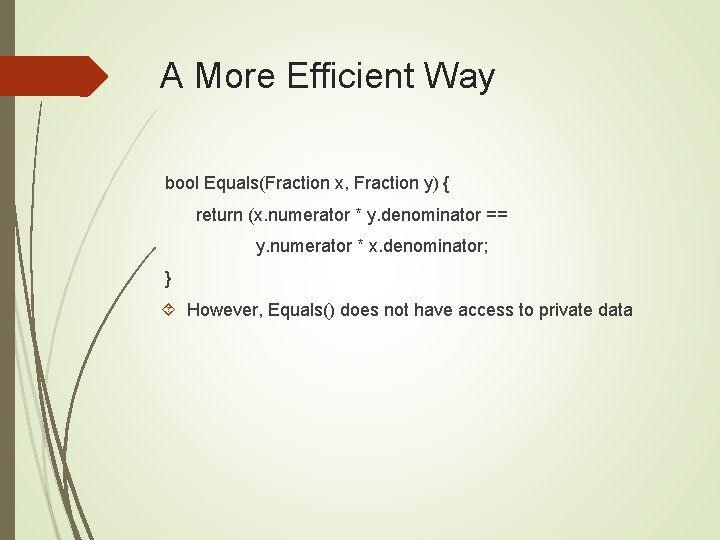A More Efficient Way bool Equals(Fraction x, Fraction y) { return (x. numerator * y. denominator == y. numerator * x. denominator; } However, Equals() does not have access to private data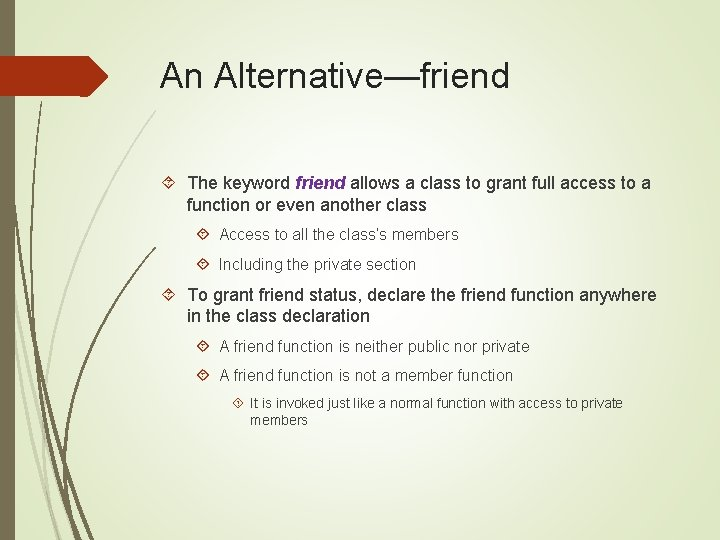An Alternative—friend The keyword friend allows a class to grant full access to a function or even another class Access to all the class’s members Including the private section To grant friend status, declare the friend function anywhere in the class declaration A friend function is neither public nor private A friend function is not a member function It is invoked just like a normal function with access to private membersExample Fraction Class http: //www. cs. fsu. edu/~myers/cop 3330/examples/class 2/fra c 1/ Equals() friend function Add() friend function A driver functionfrac. h class Fraction { friend bool Equals(Fraction x, Fraction y); friend Fraction Add(Fraction x, Fraction y); public: … private: … };frac. cpp Note: no keyword friend here #include <iostream> … bool Equals(Fraction x, Fraction y) { return (x. numerator * y. denominator == y. numerator * x. denominator); } Note: no keyword friend here Fraction Add(Fraction x, Fraction y) { int num = x. numerator * y. denominator + y. numberator * x. denominator; int denom = x. denominator * y. denominator; return Fraction(num, denom); } …driver. cpp #include <iostream> #include “frac. h” using namespace std; int main() { Fraction f 1, f 2, f 3, f 4 cout << “Enter fraction f 1: “; f 1. Input(); cout << “Enter fraction f 2: “; f 2. Input(); cout << “nf 1 = “; f 1. Show(); cout << “nf 2 = “; f 2. Show(); cout << “nn”;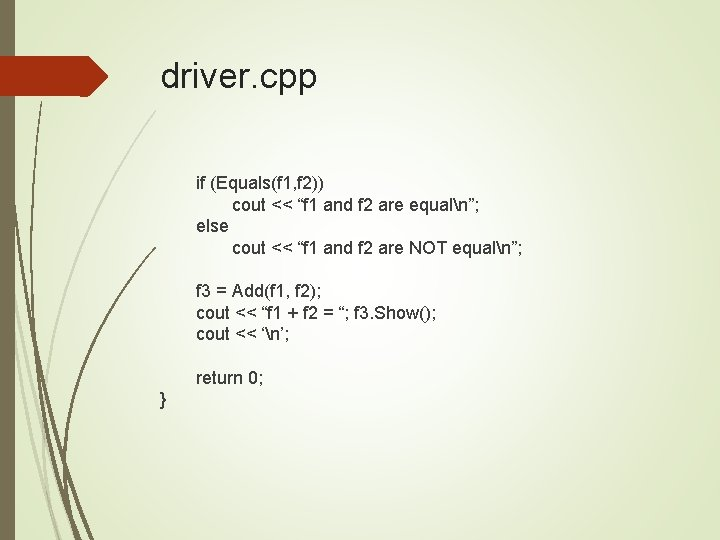driver. cpp if (Equals(f 1, f 2)) cout << “f 1 and f 2 are equaln”; else cout << “f 1 and f 2 are NOT equaln”; f 3 = Add(f 1, f 2); cout << “f 1 + f 2 = “; f 3. Show(); cout << ‘n’; return 0; }Another Option Use a member function if (f 1. Equals(f 2)) cout << “The fractions are equaln”; f 1 is a calling object f 2 is a parameter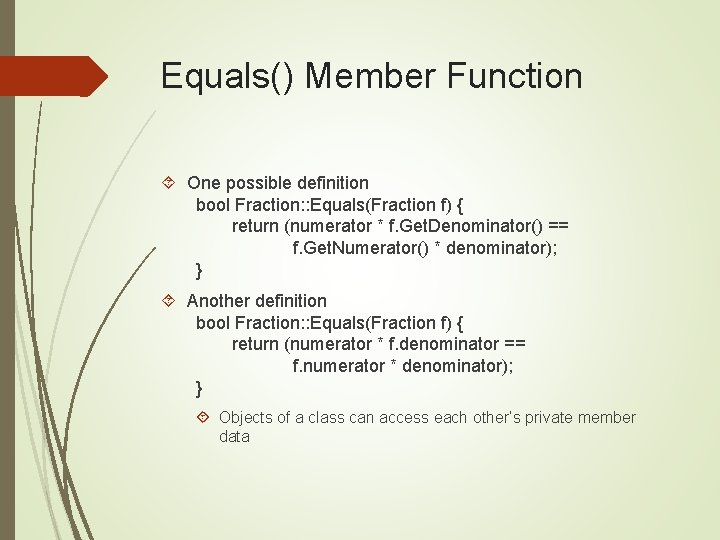Equals() Member Function One possible definition bool Fraction: : Equals(Fraction f) { return (numerator * f. Get. Denominator() == f. Get. Numerator() * denominator); } Another definition bool Fraction: : Equals(Fraction f) { return (numerator * f. denominator == f. numerator * denominator); } Objects of a class can access each other’s private member data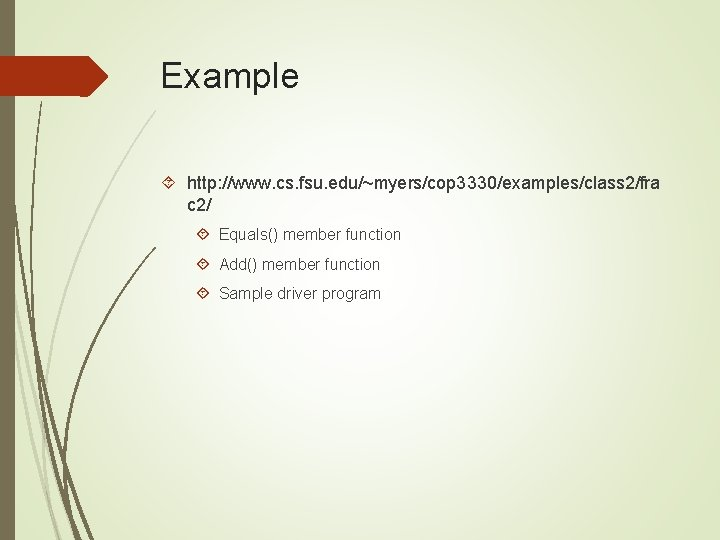Example http: //www. cs. fsu. edu/~myers/cop 3330/examples/class 2/fra c 2/ Equals() member function Add() member function Sample driver programfrac. h class Fraction { public: … bool Equals(Fraction f); Fraction Add(Fraction f); private: … };frac. cpp include <iostream> … bool Fraction: : Equals(Fraction f) { return (numerator * f. denominator == f. numerator * denominator); } Fraction: : Add(Fraction f) { int num = numerator * f. denominator + f. numberator * denominator; int denom = denominator * f. denominator; return Fraction(num, denom); } …driver. cpp #include <iostream> #include “frac. h” using namespace std; int main() { Fraction f 1, f 2, f 3, f 4; cout << “Enter fraction f 1: “; f 1. Input(); cout << “Enter fraction f 2: “; f 2. Input(); cout << “nf 1 = “; f 1. Show(); cout << “nf 2 = “; f 2. Show(); cout << “nn”;driver. cpp if (f 1. Equals(f 2)) cout << “f 1 and f 2 are equaln”; else cout << “f 1 and f 2 are NOT equaln”; f 3 = f 1. Add(f 2); cout << “f 1 + f 2 = “; f 3. Show(); cout << ‘n’; return 0; }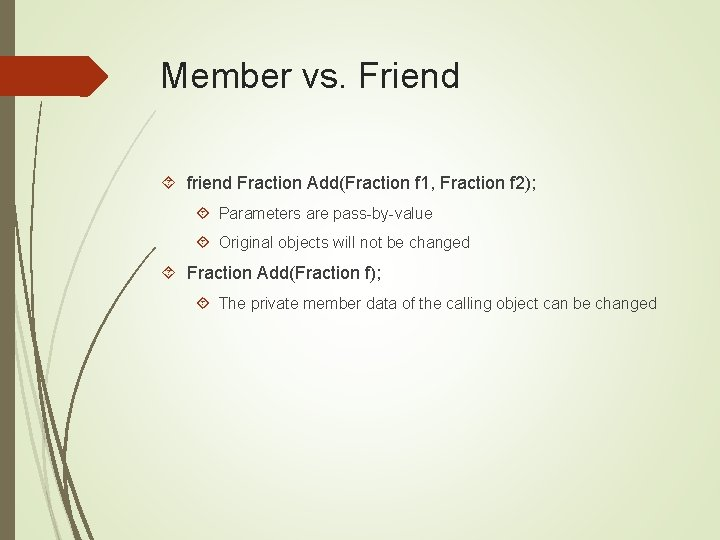Member vs. Friend friend Fraction Add(Fraction f 1, Fraction f 2); Parameters are pass-by-value Original objects will not be changed Fraction Add(Fraction f); The private member data of the calling object can be changed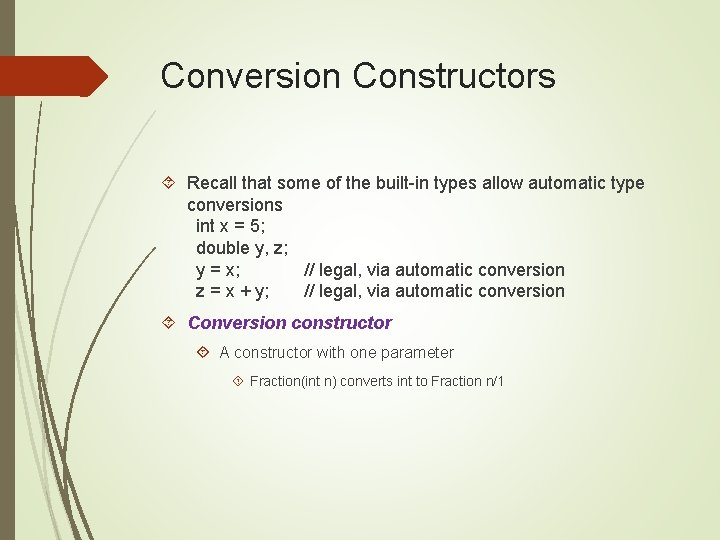Conversion Constructors Recall that some of the built-in types allow automatic type conversions int x = 5; double y, z; y = x; // legal, via automatic conversion z = x + y; // legal, via automatic conversion Conversion constructor A constructor with one parameter Fraction(int n) converts int to Fraction n/1Explicit and Implicit Conversions Fraction f 1, f 2 // create fraction objects; f 1 = Fraction(4); // explicit call to conversion constructor f 2 = 10; // implicit call to conversion constructor // equivalent to f 2 = Fraction(10) f 1 = Add(f 2, 5); // implicitly converts 5 to Fraction 5/1 Fraction(int n, int d = 1) counts as a conversion constructor Since the second parameter is optional Suppress implicit conversion explicit Fraction(double d); // will NOT be used for // automatic conversions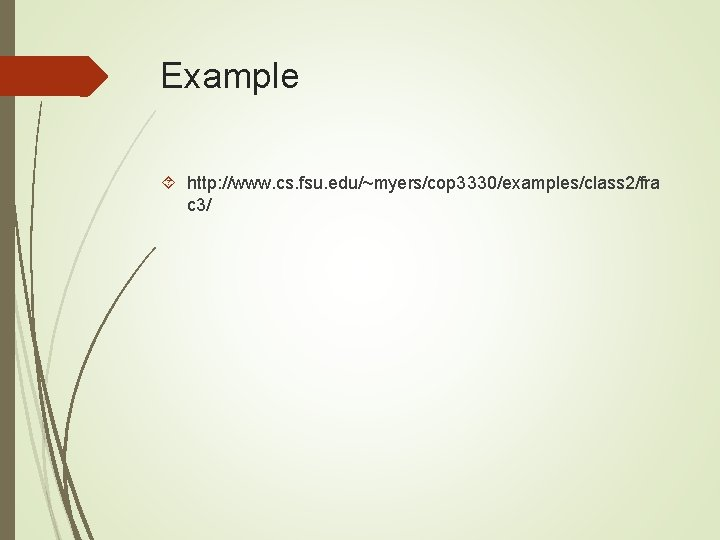Example http: //www. cs. fsu. edu/~myers/cop 3330/examples/class 2/fra c 3/frac. h class Fraction { public: … Fraction(int n, int d=1); explicit Fraction(double d); … private: … };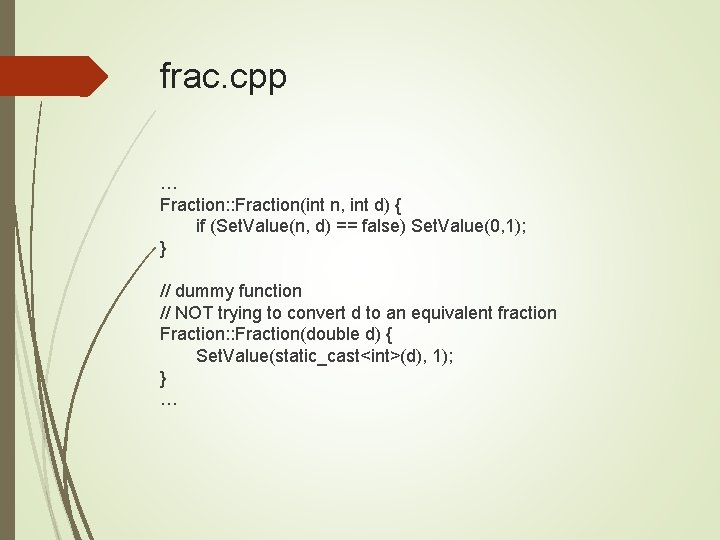frac. cpp … Fraction: : Fraction(int n, int d) { if (Set. Value(n, d) == false) Set. Value(0, 1); } // dummy function // NOT trying to convert d to an equivalent fraction Fraction: : Fraction(double d) { Set. Value(static_cast<int>(d), 1); } …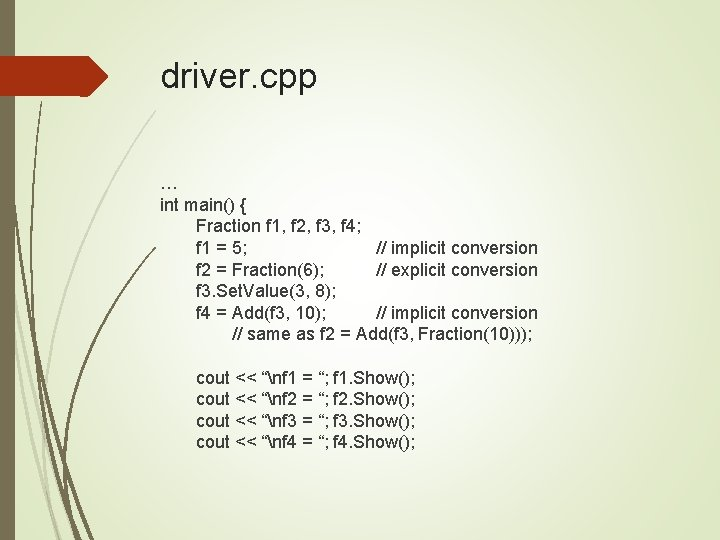driver. cpp … int main() { Fraction f 1, f 2, f 3, f 4; f 1 = 5; // implicit conversion f 2 = Fraction(6); // explicit conversion f 3. Set. Value(3, 8); f 4 = Add(f 3, 10); // implicit conversion // same as f 2 = Add(f 3, Fraction(10))); cout << “nf 1 = “; f 1. Show(); cout << “nf 2 = “; f 2. Show(); cout << “nf 3 = “; f 3. Show(); cout << “nf 4 = “; f 4. Show();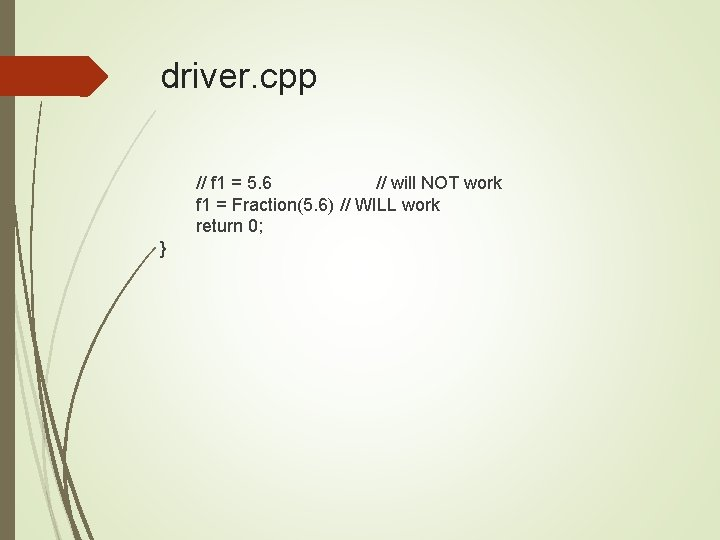driver. cpp // f 1 = 5. 6 // will NOT work f 1 = Fraction(5. 6) // WILL work return 0; }Using const in Class Specifies what is not allowed to change, within some scope Clarifies the intent of the code to other users Affects how certain items can be usedL-Value vs. R-Value L-value = r-value; x = 10 vs. 10 = x? L-value can appear on the left-hand side of an assignment R-value can appear on the right-hand side of an assignment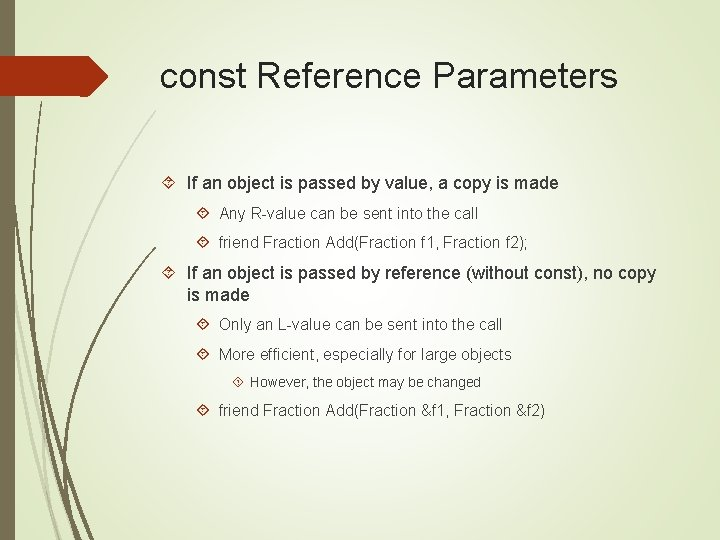const Reference Parameters If an object is passed by value, a copy is made Any R-value can be sent into the call friend Fraction Add(Fraction f 1, Fraction f 2); If an object is passed by reference (without const), no copy is made Only an L-value can be sent into the call More efficient, especially for large objects However, the object may be changed friend Fraction Add(Fraction &f 1, Fraction &f 2)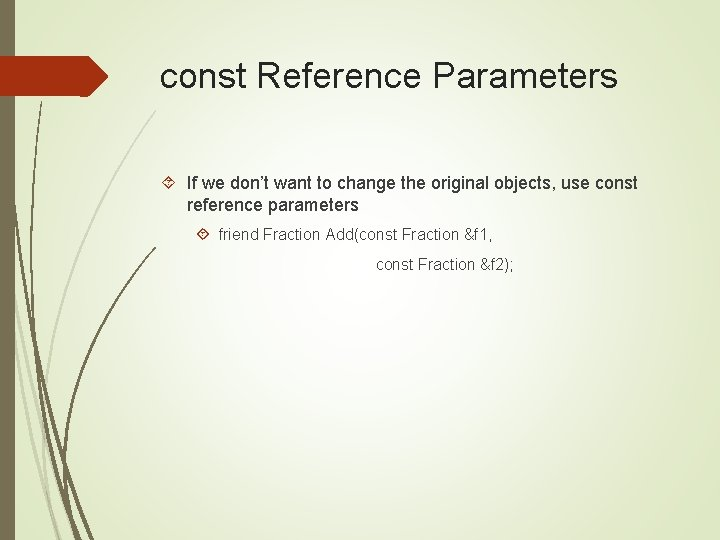const Reference Parameters If we don’t want to change the original objects, use const reference parameters friend Fraction Add(const Fraction &f 1, const Fraction &f 2);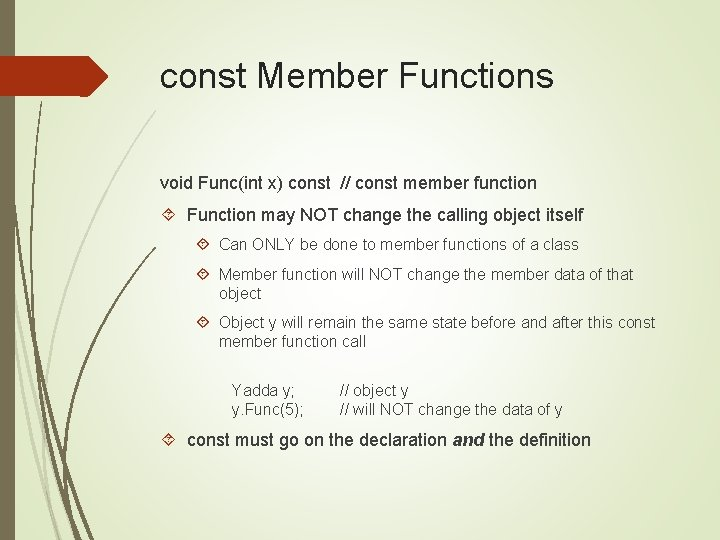const Member Functions void Func(int x) const // const member function Function may NOT change the calling object itself Can ONLY be done to member functions of a class Member function will NOT change the member data of that object Object y will remain the same state before and after this const member function call Yadda y; y. Func(5); // object y // will NOT change the data of y const must go on the declaration and the definitionconst Member Functions Should be used whenever appropriate for good class design Constructors initialize the object Would not be const functions Mutators change member data Would not be const functions Pure accessor functions retrieve member data Would typically be const functions Functions for printing (e. g. , Show(), Display()) Would be good candidates for const functions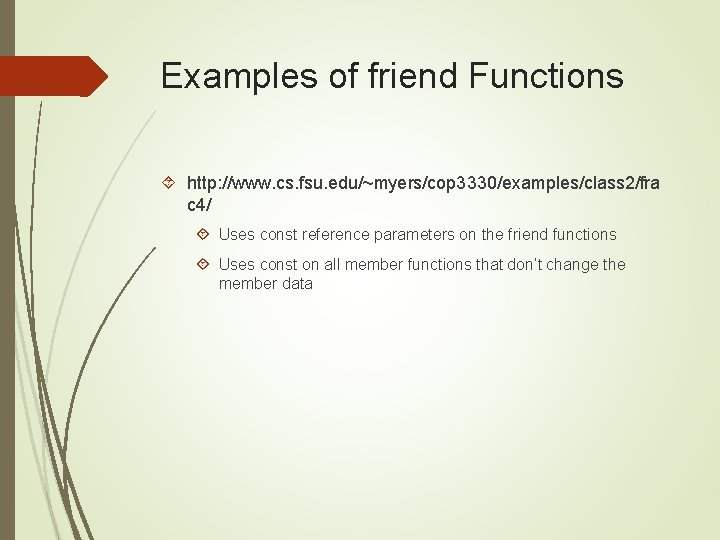Examples of friend Functions http: //www. cs. fsu. edu/~myers/cop 3330/examples/class 2/fra c 4/ Uses const reference parameters on the friend functions Uses const on all member functions that don’t change the member datafrac. h class Fraction { friend bool Equals(const Fraction &x, const Fraction &y); friend Fraction Add(const Fraction &x, const Fraction &y); public: Fraction(); Fraction(int n, int d=1); void Input(); void Show() const; int Get. Numerator() const; int Get. Denominator() const; bool Set. Value(int n, int d); double Evaluate() const; private: int numerator, denominator; };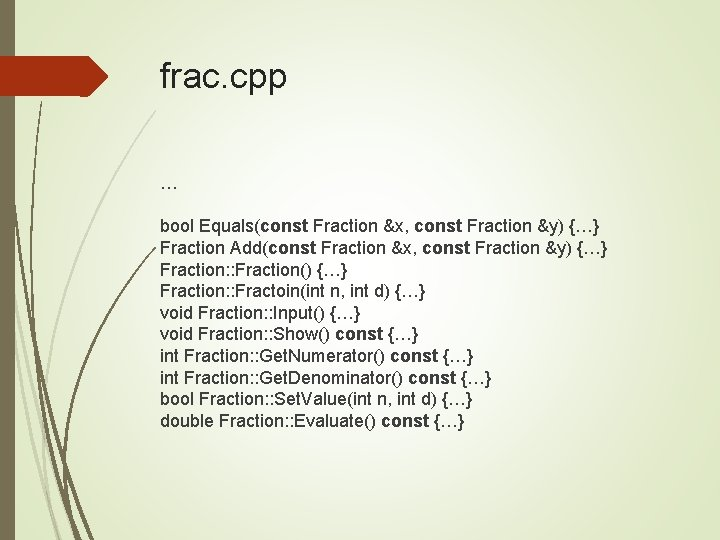frac. cpp … bool Equals(const Fraction &x, const Fraction &y) {…} Fraction Add(const Fraction &x, const Fraction &y) {…} Fraction: : Fraction() {…} Fraction: : Fractoin(int n, int d) {…} void Fraction: : Input() {…} void Fraction: : Show() const {…} int Fraction: : Get. Numerator() const {…} int Fraction: : Get. Denominator() const {…} bool Fraction: : Set. Value(int n, int d) {…} double Fraction: : Evaluate() const {…}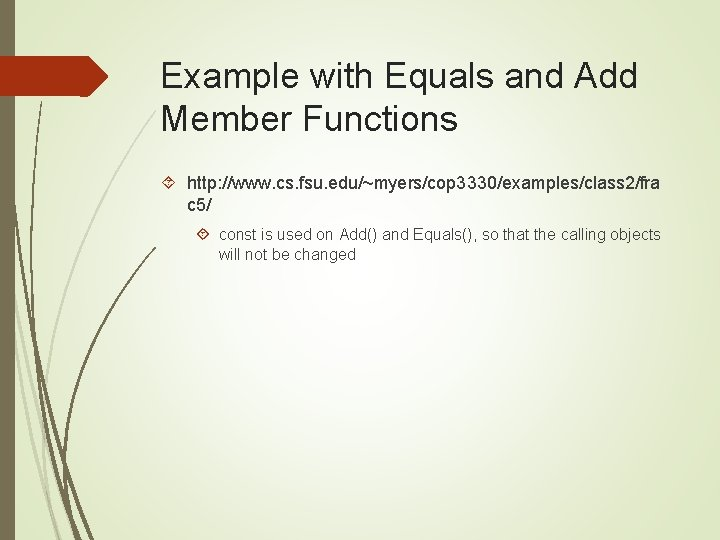Example with Equals and Add Member Functions http: //www. cs. fsu. edu/~myers/cop 3330/examples/class 2/fra c 5/ const is used on Add() and Equals(), so that the calling objects will not be changedfrac. h class Fraction { public: Fraction(); Fraction(int n, int d=1); void Input(); void Show() const; Fraction f will int Get. Numerator() const; not be int Get. Denominator() const; changed bool Set. Value(int n, int d); double Evaluate() const; bool Equals(const Fraction &f) const; Fraction Add(const Fraction &f) const; Numerator and private: denominator int numerator, denominator; will not be }; changedfrac. cpp … bool Fraction: : Equals(const Fraction &f) const {…} Fraction: : Add(const Fraction &f) const {…} Fraction: : Fraction() {…} Fraction: : Fractoin(int n, int d) {…} void Fraction: : Input() {…} void Fraction: : Show() const {…} int Fraction: : Get. Numerator() const {…} int Fraction: : Get. Denominator() const {…} bool Fraction: : Set. Value(int n, int d) {…} double Fraction: : Evaluate() const {…}Declaring const Objects Need to be initialized at the same line const int SIZE = 10; const double PI = 3. 1415; Objects can also be declared as const Constructor will be involved An object’s state cannot be changed afterwards const Fraction ZERO; // fixed at 0/1 const Fraction FIXED(3, 4); // fixed at ¾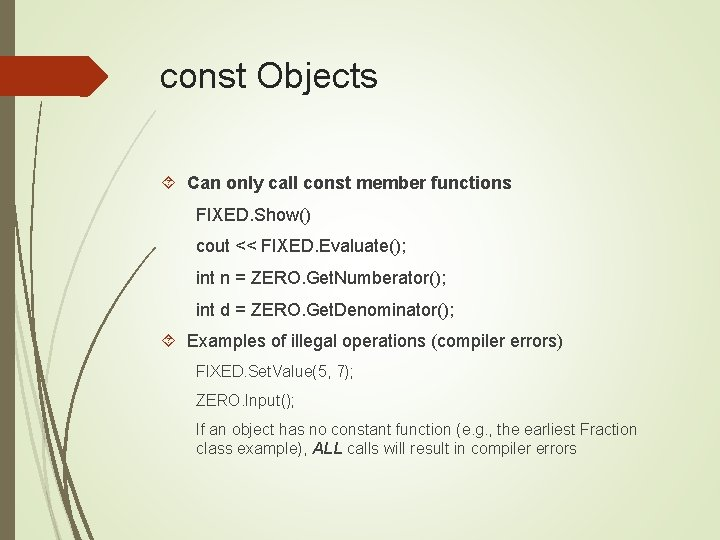const Objects Can only call const member functions FIXED. Show() cout << FIXED. Evaluate(); int n = ZERO. Get. Numberator(); int d = ZERO. Get. Denominator(); Examples of illegal operations (compiler errors) FIXED. Set. Value(5, 7); ZERO. Input(); If an object has no constant function (e. g. , the earliest Fraction class example), ALL calls will result in compiler errors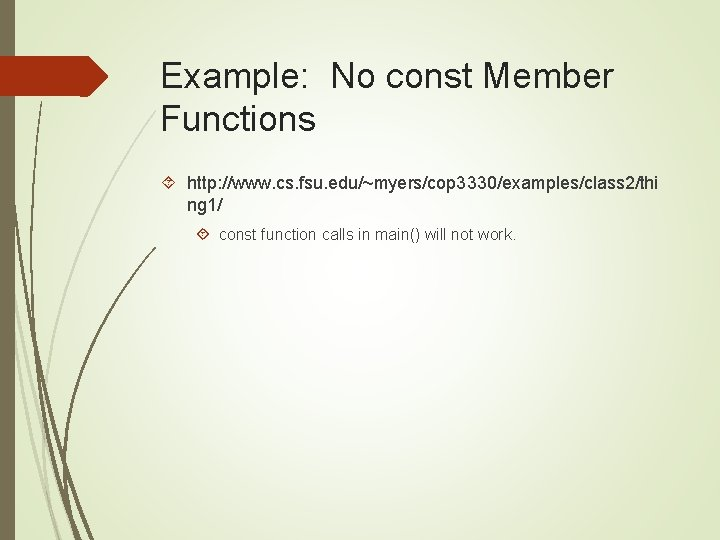Example: No const Member Functions http: //www. cs. fsu. edu/~myers/cop 3330/examples/class 2/thi ng 1/ const function calls in main() will not work.thing. h class Thing { public: Thing(); Thing(int h, int w); void Show(); void Set(int h, int w); int Get. Height(); int Get. Weight(); private: int height, weight; };thing. cpp #include <iostream> #include “thing. h” using namespace std; Thing: : Thing() { height = weight = 0; } Thing: : Thing(int h, int w) { height = h; weight = w; } void Thing: : Show() { cout << “Height = “ height << “tt. Weight = “ << weight << ‘n’; } void Thing: : Set(int h, int w) { height = h; weight = w; } Int Thing: : Get. Height() { return height; } Int Thing: : Get. Weight() { return weight; }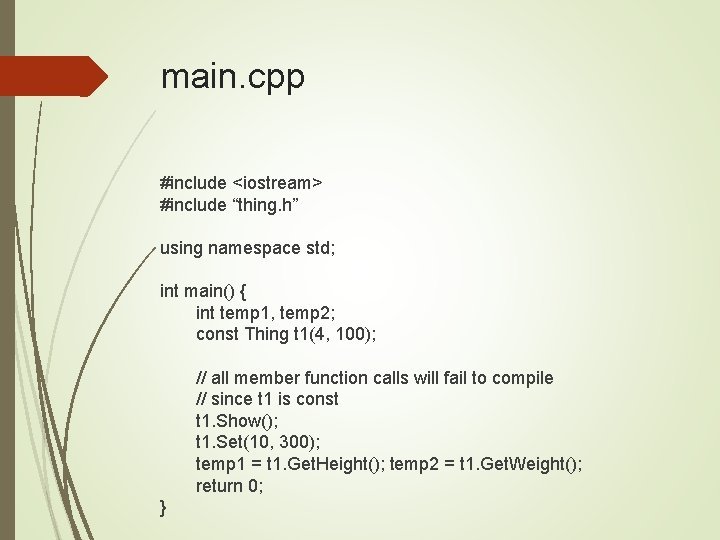main. cpp #include <iostream> #include “thing. h” using namespace std; int main() { int temp 1, temp 2; const Thing t 1(4, 100); // all member function calls will fail to compile // since t 1 is const t 1. Show(); t 1. Set(10, 300); temp 1 = t 1. Get. Height(); temp 2 = t 1. Get. Weight(); return 0; }Example: const Member Functions http: //www. cs. fsu. edu/~myers/cop 3330/examples/class 2/thi ng 2/ const member function calls will compile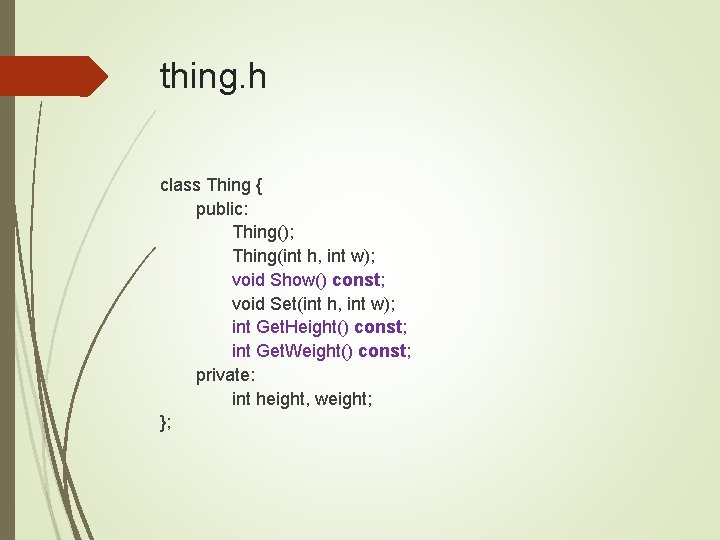thing. h class Thing { public: Thing(); Thing(int h, int w); void Show() const; void Set(int h, int w); int Get. Height() const; int Get. Weight() const; private: int height, weight; };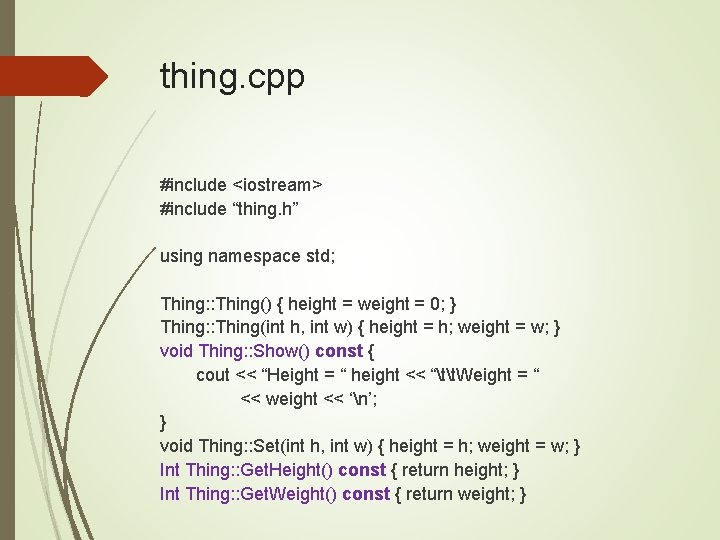thing. cpp #include <iostream> #include “thing. h” using namespace std; Thing: : Thing() { height = weight = 0; } Thing: : Thing(int h, int w) { height = h; weight = w; } void Thing: : Show() const { cout << “Height = “ height << “tt. Weight = “ << weight << ‘n’; } void Thing: : Set(int h, int w) { height = h; weight = w; } Int Thing: : Get. Height() const { return height; } Int Thing: : Get. Weight() const { return weight; }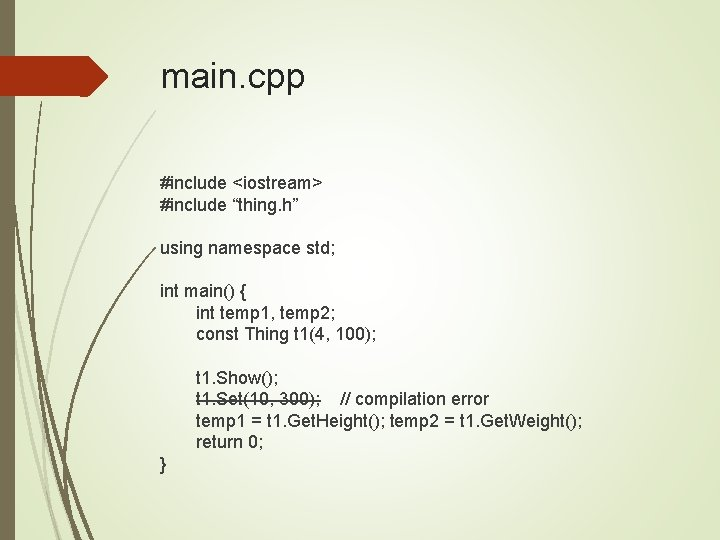main. cpp #include <iostream> #include “thing. h” using namespace std; int main() { int temp 1, temp 2; const Thing t 1(4, 100); t 1. Show(); t 1. Set(10, 300); // compilation error temp 1 = t 1. Get. Height(); temp 2 = t 1. Get. Weight(); return 0; }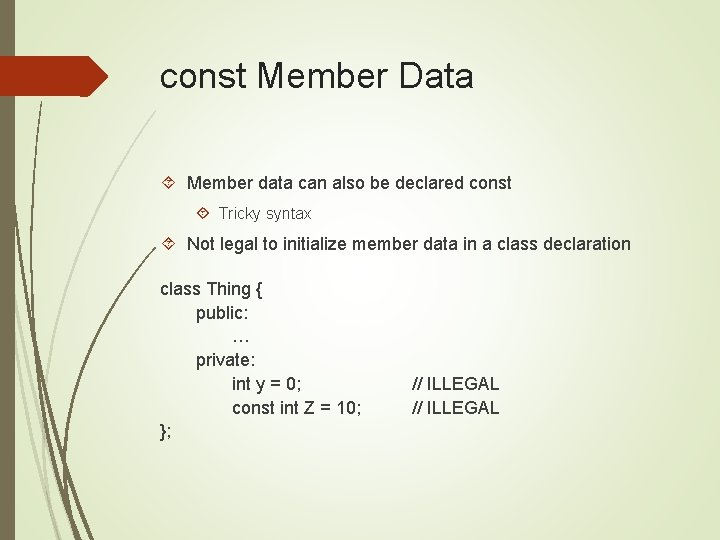const Member Data Member data can also be declared const Tricky syntax Not legal to initialize member data in a class declaration class Thing { public: … private: int y = 0; const int Z = 10; }; // ILLEGALAnother Try thing. h class Thing { public: … private: int y; const int Z; }; thing. cpp Thing: : Thing() { y = 0; Z = 10; // ILLEGAL }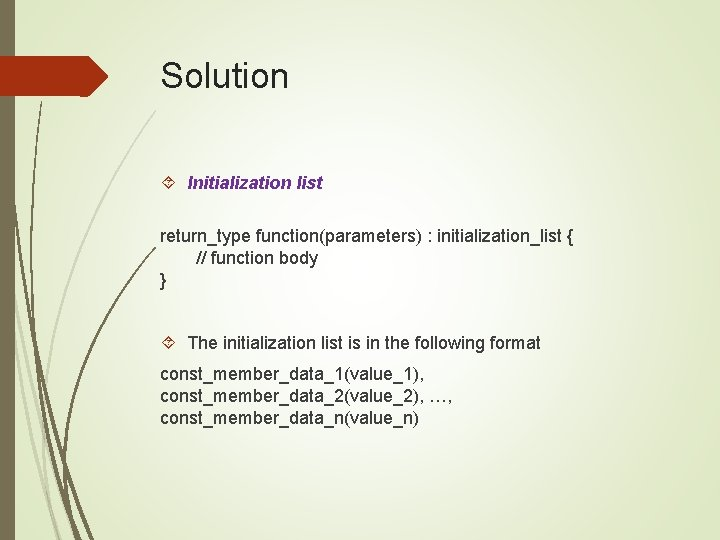Solution Initialization list return_type function(parameters) : initialization_list { // function body } The initialization list is in the following format const_member_data_1(value_1), const_member_data_2(value_2), …, const_member_data_n(value_n)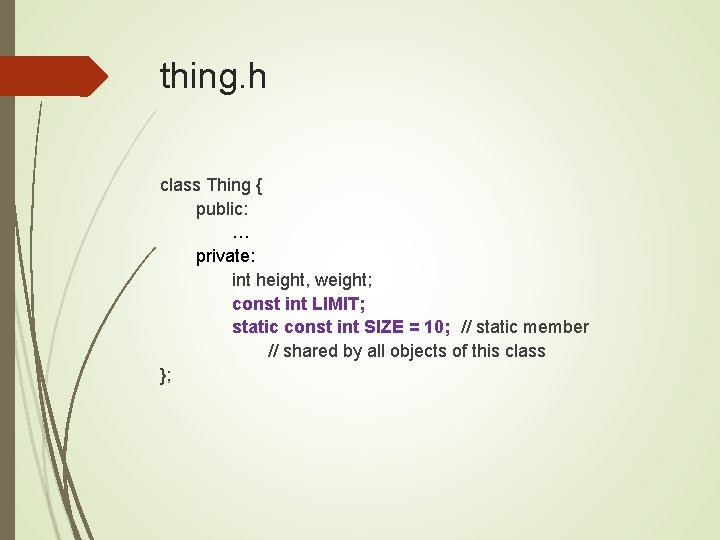thing. h class Thing { public: … private: int height, weight; const int LIMIT; static const int SIZE = 10; // static member // shared by all objects of this class };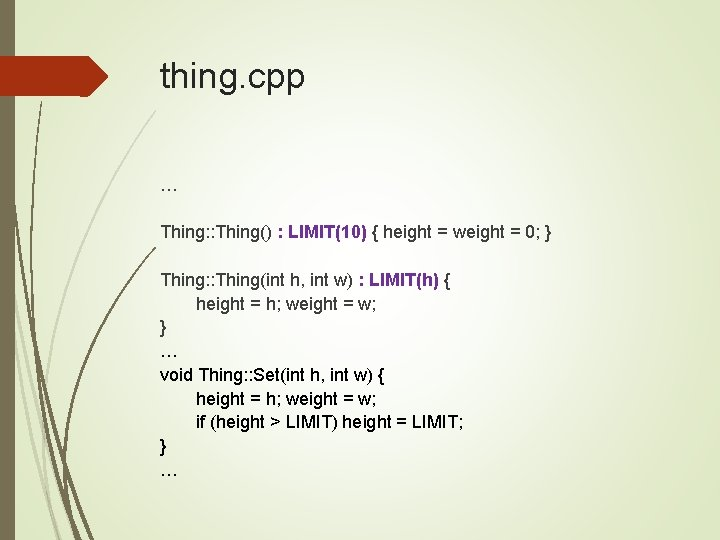thing. cpp … Thing: : Thing() : LIMIT(10) { height = weight = 0; } Thing: : Thing(int h, int w) : LIMIT(h) { height = h; weight = w; } … void Thing: : Set(int h, int w) { height = h; weight = w; if (height > LIMIT) height = LIMIT; } …main. cpp #include <iostream> #include “thing. h” using namespace std; int main() { int temp 1, temp 2; Thing t 1(12, 100); t 1. Show(); cout << “n”; t 1. Set(13, 400); t 1. Show(); cout << “n”; return 0; }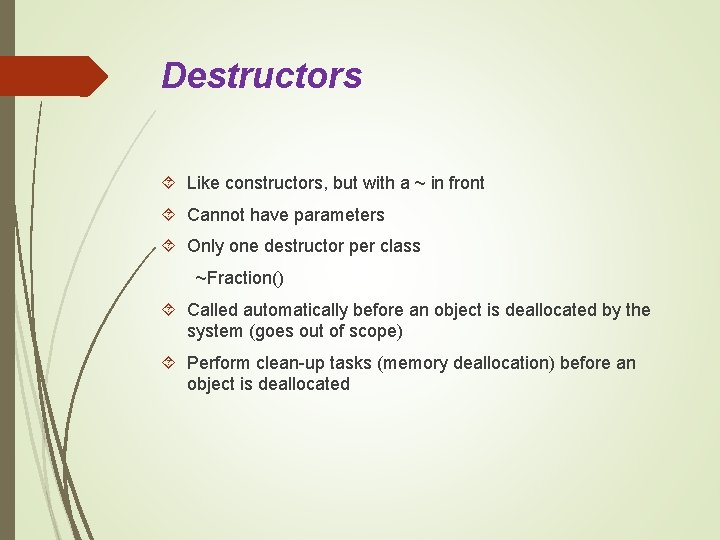Destructors Like constructors, but with a ~ in front Cannot have parameters Only one destructor per class ~Fraction() Called automatically before an object is deallocated by the system (goes out of scope) Perform clean-up tasks (memory deallocation) before an object is deallocated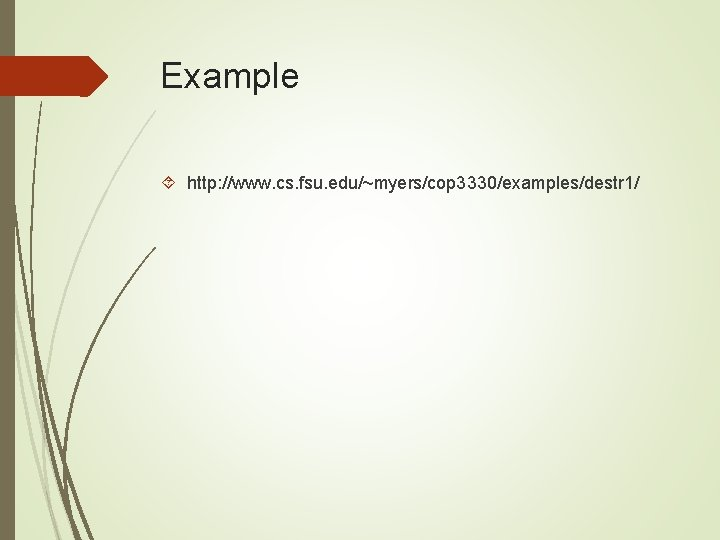Example http: //www. cs. fsu. edu/~myers/cop 3330/examples/destr 1/thing. h class Thing { public: Thing(); Thing(int x); ~Thing(); private: int data; };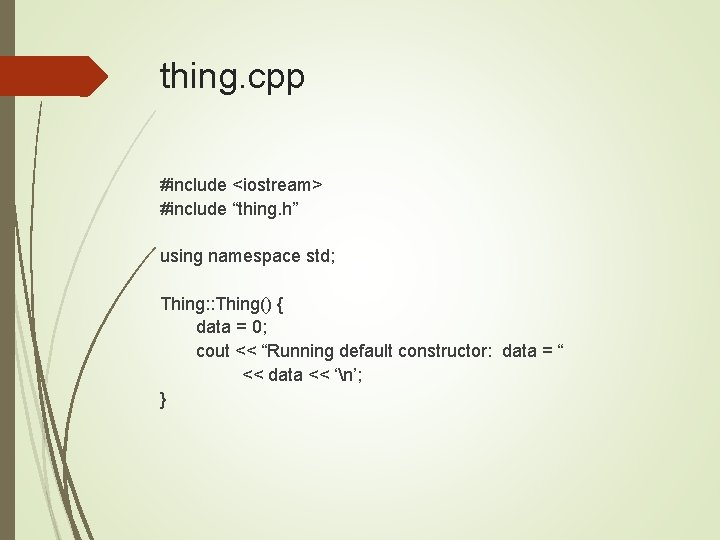thing. cpp #include <iostream> #include “thing. h” using namespace std; Thing: : Thing() { data = 0; cout << “Running default constructor: data = “ << data << ‘n’; }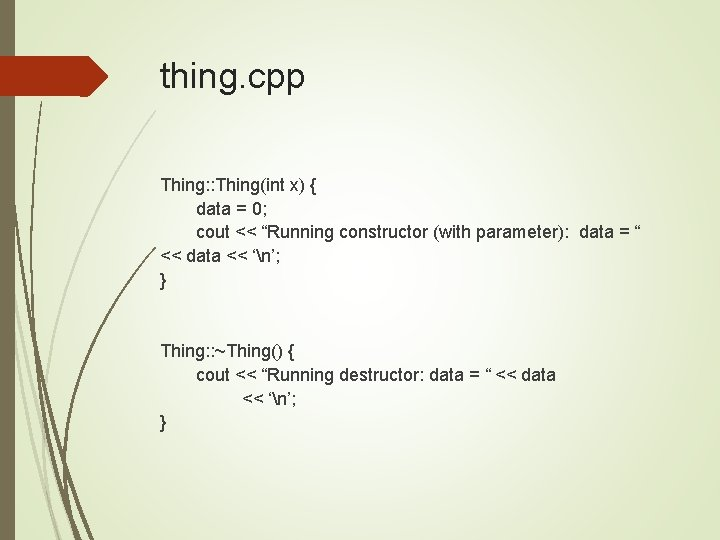thing. cpp Thing: : Thing(int x) { data = 0; cout << “Running constructor (with parameter): data = “ << data << ‘n’; } Thing: : ~Thing() { cout << “Running destructor: data = “ << data << ‘n’; }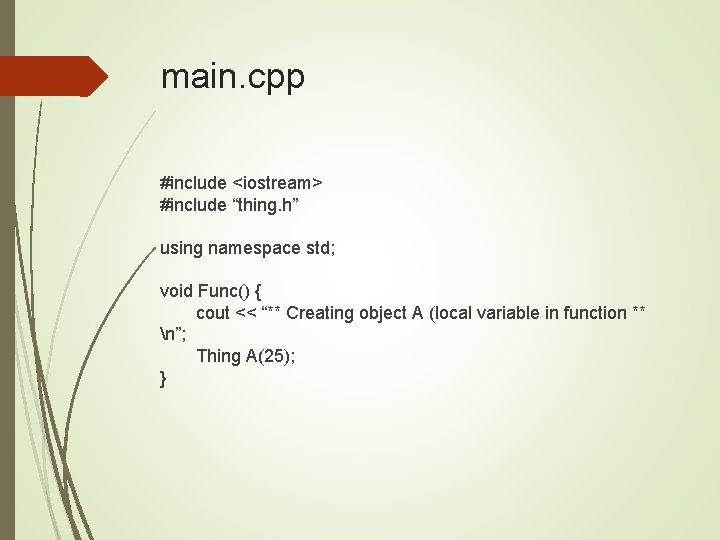main. cpp #include <iostream> #include “thing. h” using namespace std; void Func() { cout << “** Creating object A (local variable in function ** n”; Thing A(25); }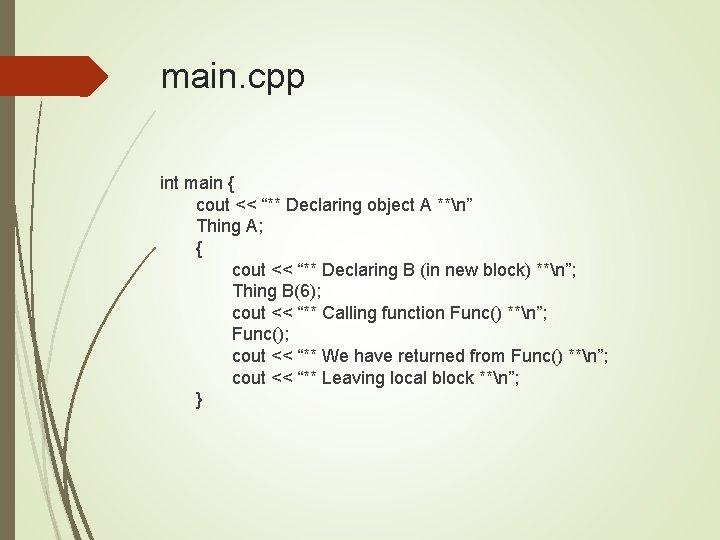main. cpp int main { cout << “** Declaring object A **n” Thing A; { cout << “** Declaring B (in new block) **n”; Thing B(6); cout << “** Calling function Func() **n”; Func(); cout << “** We have returned from Func() **n”; cout << “** Leaving local block **n”; }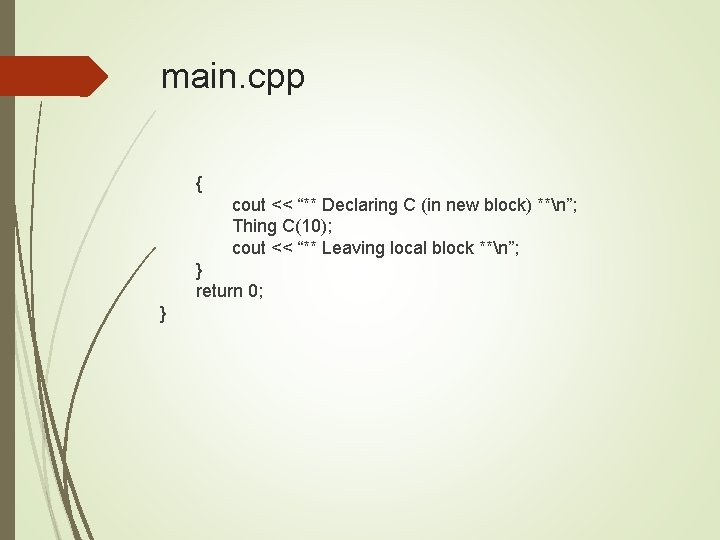main. cpp { cout << “** Declaring C (in new block) **n”; Thing C(10); cout << “** Leaving local block **n”; } return 0; }